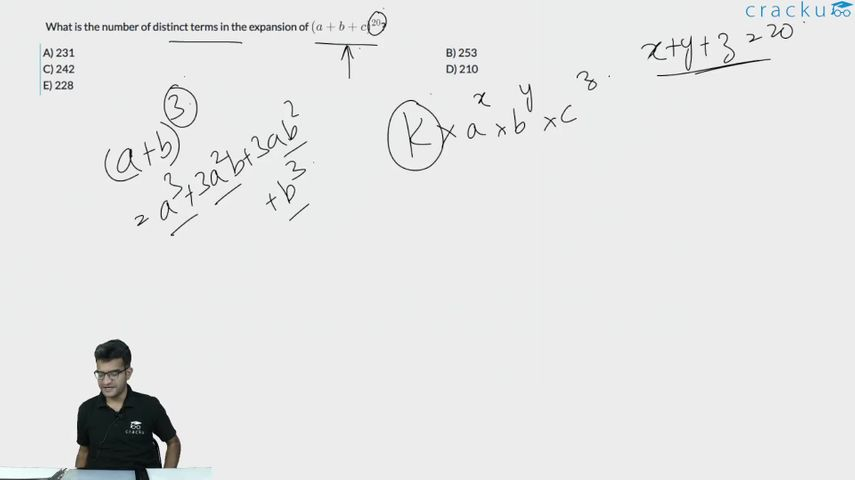Question 18

# What is the number of distinct terms in the expansion of $$(a + b + c)^{20}$$?

Solution

The power is 20.

20 has to be divided among a, b and c. This can be done in $$^{20+3-1}C_{3-1}$$ = $$^{22}C_2$$ = 231

Option a) is the correct answer.

### View Video Solution